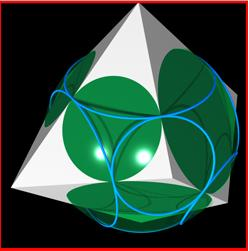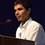# 3-D Geometry Questions(1) Find the side of a regular tetrahedron which is inside a sphere of radius ' r ', Exactly fitting.

(Just as a triangle has circumcircle, say the Tetrahedron has a Circumsphere)

(2) A solid tetrahedron is sliced off a wooden cube of side 'a' by a plane passing through two nonadjacent vertices on one face and one vertex on the opposite face not adjacent to either of the first two vertices.

The tetrahedron is discarded and the remaining portion of the cube is placed on a table with the cut surface face down.

What is the height of this object?Note by Piyushkumar Palan
6 years, 7 months ago

This discussion board is a place to discuss our Daily Challenges and the math and science related to those challenges. Explanations are more than just a solution — they should explain the steps and thinking strategies that you used to obtain the solution. Comments should further the discussion of math and science.

When posting on Brilliant:

• Use the emojis to react to an explanation, whether you're congratulating a job well done , or just really confused .
• Ask specific questions about the challenge or the steps in somebody's explanation. Well-posed questions can add a lot to the discussion, but posting "I don't understand!" doesn't help anyone.
• Try to contribute something new to the discussion, whether it is an extension, generalization or other idea related to the challenge.

MarkdownAppears as
*italics* or _italics_ italics
**bold** or __bold__ bold
- bulleted- list
• bulleted
• list
1. numbered2. list
1. numbered
2. list
Note: you must add a full line of space before and after lists for them to show up correctly
paragraph 1paragraph 2

paragraph 1

paragraph 2

[example link](https://brilliant.org)example link
> This is a quote
This is a quote
    # I indented these lines
# 4 spaces, and now they show
# up as a code block.

print "hello world"
# I indented these lines
# 4 spaces, and now they show
# up as a code block.

print "hello world"
MathAppears as
Remember to wrap math in $$ ... $$ or $ ... $ to ensure proper formatting.
2 \times 3 $2 \times 3$
2^{34} $2^{34}$
a_{i-1} $a_{i-1}$
\frac{2}{3} $\frac{2}{3}$
\sqrt{2} $\sqrt{2}$
\sum_{i=1}^3 $\sum_{i=1}^3$
\sin \theta $\sin \theta$
\boxed{123} $\boxed{123}$

Sort by:

A better description of the problem is a plane passing through all the vertexes connected to a vertex by sides.

The diagonal of this cube is $sqrt{2}$ a which we shall call b for simplicity.

Now we have a tetrahedron with base length of b and side length of a

Now we proceed to calculate the height of this shape:

1) the base of the triangle:

Imagine a top view of the tetrahedron, an equilateral triangle split into three equal isosceles triangles (sides b, c and c). (30, 30, 120 degrees) (as they bisect the 60 degree angle of the equilateral triangle)

First we find the length from the mid point of b to the centre of the triangle. (cosine rule works) but i shall use area for simplicity.

Area of equilateral triangle is (b^2 root(3))/4

area of one isosceles is one third this (b^2 root(3))/12

area is also half bc sin(30)

solve for c and you get (b root(3))/3

using pythag you get (b root(3))/6 for the distance from midpoint of b to the centre

2) the face of the triangle

the length obviously = b/2

by pythag we have the height of the tetrahedron as 2 root{2}b

pythag gives us root(2)b as the diagonal length

now the length of the diagonal - the hight of the tetrahedron will give the answer to the question, but i have made an error somewhere along the way.

i currently do not have the time to re-do but i shall do so later. sorry.

I shall post this anyway as it shows the process (minus algebraic manipulation) to reach the answer

also sorry i cannot figure out formatting and i'm pressed for time

- 6 years, 7 months ago

- 6 years, 7 months ago

thanks Felix

- 6 years, 7 months ago

2nd problem is really good I had to use the $\text{Colours}$ $\text{Cube}$ (played as a puzzle) for the imagination :P....Ans can be found by subtracting $\text{height}$ $\text{of}$ $\text{tetrahedron}$ from $\text{body}$ $\text{diagonal}$ $\text{of}$ $\text{cube}$ so ans is $\frac {2}{\sqrt{3}}a$

Worked solution...Consider the tetrahedron with vertical angle $\frac{\pi}{2}$ steradian (angle at vertex of cube) and base equilateral triangle of side $\sqrt{2} a$ (which is face diagonal of cube )............This equilateral triangle has $C$ as circumcentre and it's circumradius will be $\frac{2}{\sqrt{3}} (\frac {1}{2} \sqrt{2}a)$= $\frac{2}{\sqrt{3}} \frac {a}{\sqrt{2}}$=$\sqrt{\frac{2}{3}}a$

Then apical vertex $A$ joined to $C$ say circumcentre(=centroid=incentre) of base will be height of tetrahedron .

In the right angled $\Delta ABC$ formed by joining a base vertex say $B$ , apical vertex $A$ and $C$ circumcentre of base, The hypotenuse is $AB$ of length 'a' which was side of cube. The segment $BC$ is of length circumradius of base=$\sqrt{\frac{2}{3}}a$ so $AC$ (height of Tetrahedron) is $\sqrt{a^2 - \frac {2}{3}a^2}$=$\frac{a}{\sqrt{3}}$ by Pythagoras' theorem

As the body diagonal of Cube is $\sqrt{3} a$ and height of Tetrahedron is $\frac{a}{\sqrt{3}}$,

The answer is $a (\sqrt{3}-\frac{1}{\sqrt{3}})$= $\frac {2}{\sqrt{3}}a$

Actually this is perhaps the same way which $Felix$ wanted to say but i hadn't read it before so didn't post as reply to $Felix$

- 6 years, 7 months ago

I feel 1st one will be easy if someone tells what is the formula for Height of a regular tetrahedron.......

- 6 years, 7 months ago

For question 1 the centre of the tetrahedron will also the the centre of the sphere find the point then the distance from this point to the vertex will be the spheres radius (or work backwards from this length if you find reverse proofs easier)

- 6 years, 7 months ago

What is 2d and 3d geometry?

- 6 years ago